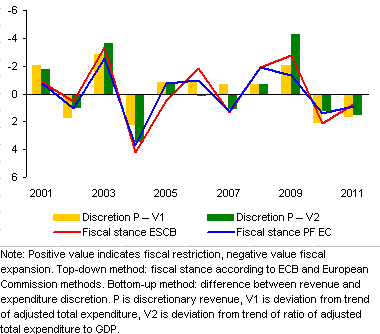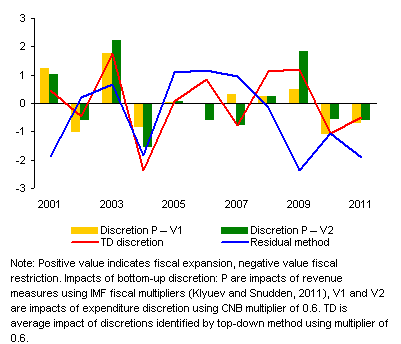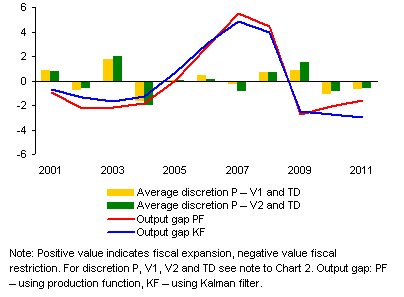# An analysis of the impacts of fiscal measures in the Czech Republic in 2001–2011

This box examines discretionary Czech fiscal policy measures in 2011–2011, analyses their economic impacts and assesses their effect on the business cycle.

Given the difficulty in distinguishing between discretionary and autonomous government revenue and expenditure, three methods of estimating fiscal discretion are used. The first, direct (“bottom-up”) method sums the impacts of individual fiscal measures on the revenue and expenditure sides of the public budgets. Explanatory reports for passed laws and regulations are the source of information for revenue measures. Expenditure discretion is proxied by the deviation of expenditure (excluding unemployment benefits, interest payments and capital transfers to transformation institutions) from “autonomous expenditure” (modelled as the trend in this expenditure or as the trend in this expenditure relative to GDP).

The second, indirect (“top-down”) method measures fiscal discretion from the aggregate perspective as the annual change in the cyclically adjusted (structural) balance of the public budgets (the “fiscal stance”) calculated according to the ESCB and European Commission methods.1 Chart 1 shows fiscal discretion estimated using the first two methods.

Chart 1 (Box)  "Bottom-up" and "top-down" fiscal discretion
The estimation of discretion by different methods yields similar results
(% of nominal GDP)The third, “residual” method measures the impact of fiscal measures on the real economy implicitly. This method uses the residual of the output gap equation of the former CNB core prediction model (QPM), taking into account the standard model demand factors.2 This method identifies demand shocks mostly in line with the previous two methods, with the significant exception of the crisis year 2009.

The impact of fiscal discretion on GDP is calculated using fiscal multipliers estimated by various authors (see Chart 2). The average impacts of fiscal discretion on GDP according to both the bottom-up and top-down methods tell qualitatively the same story (see Chart 3). The impact of the 2009 anti-crisis package is estimated at 0.9–1.5% of GDP, in 2010 the impact of discretion was between -0.8% and -1.0% of GDP due to the austerity package, and in 2011 the impact of further consolidation on GDP is estimated at -0.5% to -0.6% of GDP.

Chart 2 (Box)  The impact of fiscal discretion on GDP
Fiscal discretion had significant impacts on the real economy in several years
(% of real GDP)Chart 3 (Box)  The output gap and the impact of fiscal discretion on GDP
Fiscal policy was mostly pro-cyclical and was counter-cyclical in only some years
(% of real GDP)The assessment of the stabilisation effect of fiscal discretion on the business cycle is performed by comparing the impacts of fiscal discretion on GDP with the output gap estimates. Fiscal measures stabilise the economy if the contribution of fiscal discretion is negatively correlated with the output gap. As Chart 3 shows, the periods of desirable counter-cyclical fiscal policy are relatively short (only 2001, 2003, 2007 and 2009), while the periods of pro-cyclical fiscal policy are dominant and longer-lasting (2002, 2004–2006, 2008, 2010–2011).

1 The fiscal position is calculated using data as of 11 December 2011. For a more detailed description of the methodology, see for example Box 3 in Inflation Report III/2009

2The QPM model is described in Coats, W., Laxton, D., Rose, D. (2003): The Czech National Bank’s Forecasting and Policy Analysis System (pdf, 4 MB)